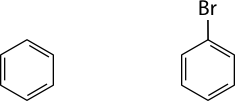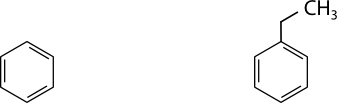# 25.15: Substitution Reactions

Organic compounds can be used for a wide variety of applications. One organic compound is halothane, an alkyl halide. This material is used as a general anesthetic for surgical procedures. When it was first developed in 1956, it was very popular, but has had limited use more recently because of toxicity issues, especially liver damage. Halothane is now used mainly as an anesthetic for children since the risk of harm is much less in these patients.

## Substitution Reactions

A substitution reaction is a reaction in which one or more atoms replace another atom or group of atoms in a molecule. Alkyl halides are formed by the substitution of a halogen atom for a hydrogen atom. When methane reacts with chlorine gas, ultraviolet light can act as a catalyst for the reaction.

$\ce{CH_4} \left( g \right) + \ce{Cl_2} \left( g \right) \overset{\text{UV light}}{\rightarrow} \ce{CH_3Cl} \left( g \right) + \ce{HCl} \left( g \right)$

The reaction produces chloromethane and hydrogen chloride. When the mixture is allowed to react for longer periods of time further substitution reactions may occur.

$\ce{CH_3Cl} \left( g \right) + \ce{Cl_2} \left( g \right) \overset{\text{UV light}}{\rightarrow} \ce{CH_2Cl_2} \left( g \right) + \ce{HCl} \left( g \right)$

The product above is dichloromethane. Further substitution produces trichloromethane and tetrachloromethane, commonly called carbon tetrachloride. A mixture of products occurs in the reaction, with the relative amounts dependent upon the time that the reaction is allowed to proceed. Chlorofluorocarbons are produced by reacting chloroalkanes with hydrofluoric acid $$\left( \ce{HF} \right)$$ because the fluorine atom bonds preferentially to the carbon.

$\ce{CCl_4} \left( g \right) + \ce{HF} \left( g \right) \overset{\ce{FeBr_3}}{\rightarrow} \ce{CCl_3F} \left( g \right) + \ce{HCl} \left( g \right)$

The fluorine atom substitutes for a chlorine atom in the reaction.

Benzene is a fairly stable and unreactive molecule due to the electron delocalization around the six-membered ring. The treatment of benzene with a halogen in the presence of an iron catalyst causes the substitution of a halogen atom for a hydrogen atom. The resulting molecule is called an aryl halide.

$\ce{C_6H_6} \left( l \right) + \ce{Br_2} \left( l \right) \overset{\ce{FeBr_3}}{\rightarrow} \ce{C_6H_5Br} \left( l \right) + \ce{HBr} \left( g \right)$Alkyl groups can be introduced onto a benzene ring by the reaction of benzene with an alkyl halide, using aluminum chloride as the catalyst. In the reaction below, benzene reacts with chloroethane to produce ethylbenzene.

$\ce{C_6H_6} \left( l \right) + \ce{CH_3CH_2Cl} \left( g \right) \overset{\ce{AlCl_3}}{\rightarrow} \ce{C_6H_5CH_2CH_3} \left( l \right) + \ce{HCl} \left( g \right)$The reaction of an alkyl halide with an inorganic hydroxide base at elevated temperature produces an alcohol. The molecular reaction to produce methanol from iodomethane and sodium hydroxide is shown below.

$\ce{CH_3I} \left( l \right) + \ce{NaOH} \left( aq \right) \overset{100^\text{o} \text{C}}{\rightarrow} \ce{CH_3OH} \left( l \right) + \ce{NaI} \left( aq \right)$

## Contributors

• CK-12 Foundation by Sharon Bewick, Richard Parsons, Therese Forsythe, Shonna Robinson, and Jean Dupon.